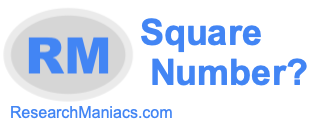Is 3 a square number?Here we will answer the question: "Is 3 a square number?" You could also ask "Is 3 a perfect square?" Square number and perfect square mean the same thing.

Anyway, for 3 to be a square number, 3 must be the product of an integer multiplied by itself. In other words, the square root of 3 must equal a whole number (integer).

There is no integer that you can multiply by itself that will make 3. Furthermore, the square root of 3 is not an integer. Thus, 3 is NOT a square number.

The answer to the question: "Is 3 a square number?" is No.
The answer to the question: "Is 3 a perfect square?" is also No.

Square Number?
Enter another number to see if it is a square number:

Is 4 a square number?
Find out if the next number on our list is a perfect square.